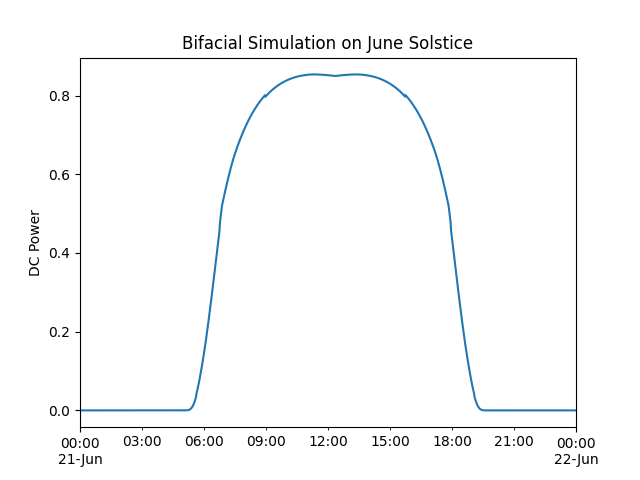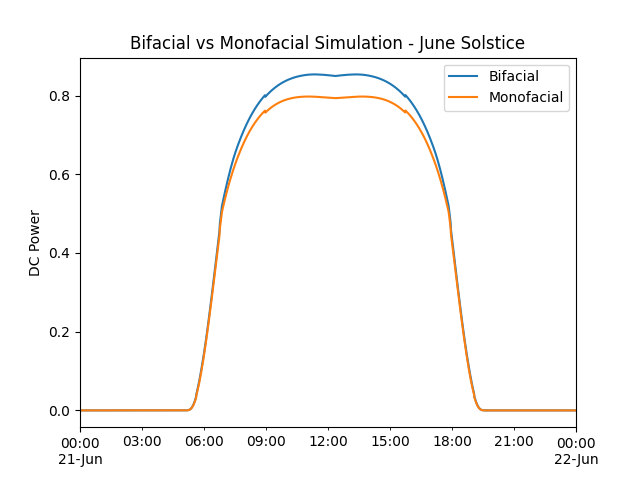# Bifacial Modeling - procedural#

Example of bifacial modeling using pvfactors and procedural method

This example shows how to complete a bifacial modeling example using the `pvlib.pvsystem.pvwatts_dc()` with the `pvlib.bifacial.pvfactors.pvfactors_timeseries()` function to transpose GHI data to both front and rear Plane of Array (POA) irradiance.

Attention

To run this example, the `solarfactors` package (an implementation of the pvfactors model) must be installed. It can be installed with either `pip install solarfactors` or `pip install pvlib[optional]`, which installs all of pvlib’s optional dependencies.

```import pandas as pd
from pvlib import location
from pvlib import tracking
from pvlib.bifacial.pvfactors import pvfactors_timeseries
from pvlib import temperature
from pvlib import pvsystem
import matplotlib.pyplot as plt
import warnings

# supressing shapely warnings that occur on import of pvfactors
warnings.filterwarnings(action='ignore', module='pvfactors')

# using Greensboro, NC for this example
lat, lon = 36.084, -79.817
tz = 'Etc/GMT+5'
times = pd.date_range('2021-06-21', '2021-06-22', freq='1T', tz=tz)

# create location object and get clearsky data
site_location = location.Location(lat, lon, tz=tz, name='Greensboro, NC')
cs = site_location.get_clearsky(times)

# get solar position data
solar_position = site_location.get_solarposition(times)

# set ground coverage ratio and max_angle to
# pull orientation data for a single-axis tracker
gcr = 0.35
max_phi = 60
orientation = tracking.singleaxis(solar_position['apparent_zenith'],
solar_position['azimuth'],
max_angle=max_phi,
backtrack=True,
gcr=gcr
)

# set axis_azimuth, albedo, pvrow width and height, and use
# the pvfactors engine for both front and rear-side absorbed irradiance
axis_azimuth = 180
pvrow_height = 3
pvrow_width = 4
albedo = 0.2

# explicity simulate on pvarray with 3 rows, with sensor placed in middle row
# users may select different values depending on needs
solar_position['apparent_zenith'],
orientation['surface_azimuth'],
orientation['surface_tilt'],
axis_azimuth,
cs.index,
cs['dni'],
cs['dhi'],
gcr,
pvrow_height,
pvrow_width,
albedo,
n_pvrows=3,
index_observed_pvrow=1
)

# turn into pandas DataFrame

# using bifaciality factor and pvfactors results, create effective irradiance
bifaciality = 0.75
* bifaciality)

# get cell temperature using the Faiman model
wind_speed=1)

# using the pvwatts_dc model and parameters detailed above,
# set pdc0 and return DC power for both bifacial and monofacial
pdc0 = 1
gamma_pdc = -0.0043
temp_cell,
pdc0,
gamma_pdc=gamma_pdc
).fillna(0)
pdc_bifi.plot(title='Bifacial Simulation on June Solstice', ylabel='DC Power')
``````<AxesSubplot:title={'center':'Bifacial Simulation on June Solstice'}, ylabel='DC Power'>
```

For illustration, perform monofacial simulation using pvfactors front-side irradiance (AOI-corrected), and plot along with bifacial results.

```effective_irrad_mono = irrad['total_abs_front']
temp_cell,
pdc0,
gamma_pdc=gamma_pdc
).fillna(0)

# plot monofacial results
plt.figure()
plt.title('Bifacial vs Monofacial Simulation - June Solstice')
pdc_bifi.plot(label='Bifacial')
pdc_mono.plot(label='Monofacial')
plt.ylabel('DC Power')
plt.legend()
# sphinx_gallery_thumbnail_number = 2
``````<matplotlib.legend.Legend object at 0x7fd4753fffd0>
```

Total running time of the script: (0 minutes 0.864 seconds)

Gallery generated by Sphinx-Gallery